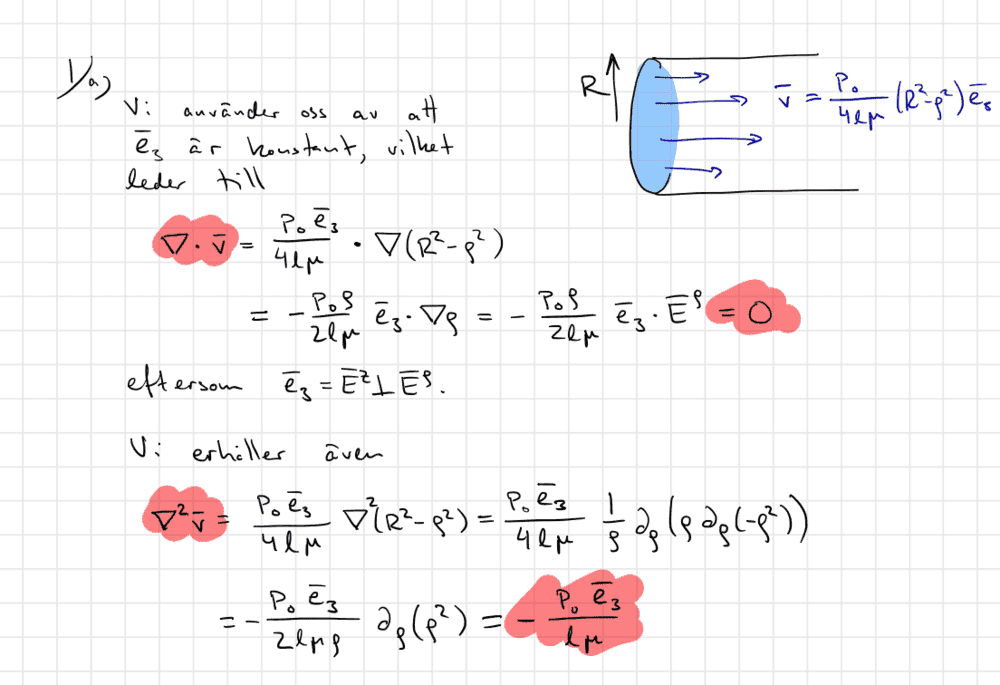# Nabla operations, vector calculus problem

• Karl Karlsson

#### Karl Karlsson

Homework Statement
A liquid flows in a cylindrical tube with the z-axis as the axis of symmetry and radius R. The flow rate of the liquid is given by $$\vec v = \frac {p_0} {4lμ} (R^2-ρ^2)$$
where ##\frac {p_0} {l}## is the pressure drop in the pipe per unit length, ##μ## is the viscosity of the liquid and ##ρ## is the radial cylinder coordinate. Calculate ##∇\cdot\vec v## and ##∇^2\cdot\vec v##
Relevant Equations
$$\vec v = \frac {p_0} {4lμ} (R^2-ρ^2)$$
Here is how my teacher solved this:I understand what the nabla operator does, ##∇\cdot\vec v## means that I am supposed to calculate ##\sum_{n=1}^3\frac {d\vec v} {dx_n}## where ##x_n## are cylindrical coordinates and ##\vec e_3 = \vec e_z##. I understand why ##∇\cdot\vec v = 0##, I would get the same answer as my teacher if I used ##\sum_{n=1}^3\frac {d\vec v} {dx_n}## to calculate it. However when I calculate ##∇^2\cdot\vec v## I don't get the same answer. I would get ##∇^2\cdot\vec v = (∇\cdot (∇v_1),∇\cdot (∇v_2),∇\cdot (∇v_3)) = \frac {p_0} {4lμ}\cdot (0,0, ∇\cdot (∇(R^2-ρ^2))) =\frac {p_0} {4lμ}\cdot (0,0, \frac {\partial^2 (R^2-ρ^2)} {\partial ρ^2})##. Which is not equal to what my teacher got. Did I miss something? Also could someone explain the steps he took to arrive at his answer?

Homework Statement:: A liquid flows in a cylindrical tube with the z-axis as the axis of symmetry and radius R. The flow rate of the liquid is given by $$\vec v = \frac {p_0} {4lμ} (R^2-ρ^2)$$
where ##\frac {p_0} {l}## is the pressure drop in the pipe per unit length, ##μ## is the viscosity of the liquid and ##ρ## is the radial cylinder coordinate. Calculate ##∇\cdot\vec v## and ##∇^2\cdot\vec v##
Relevant Equations:: $$\vec v = \frac {p_0} {4lμ} (R^2-ρ^2)$$

Here is how my teacher solved this:
View attachment 268604
I understand what the nabla operator does, ##∇\cdot\vec v## means that I am supposed to calculate ##\sum_{n=1}^3\frac {d\vec v} {dx_n}## where ##x_n## are cylindrical coordinates and ##\vec e_3 = \vec e_z##. I understand why ##∇\cdot\vec v = 0##, I would get the same answer as my teacher if I used ##\sum_{n=1}^3\frac {d\vec v} {dx_n}## to calculate it. However when I calculate ##∇^2\cdot\vec v## I don't get the same answer. I would get ##∇^2\cdot\vec v = (∇\cdot (∇v_1),∇\cdot (∇v_2),∇\cdot (∇v_3))
The last expression above doesn't seem right to me, but I am somewhat rusty with this stuff. The part that bothers me is where you're taking the gradient of the individual components ##v_1, v_2, v_3##.
Karl Karlsson said:
= \frac {p_0} {4lμ}\cdot (0,0, ∇\cdot (∇(R^2-ρ^2))) =\frac {p_0} {4lμ}\cdot (0,0, \frac {\partial^2 (R^2-ρ^2)} {\partial ρ^2})##. Which is not equal to what my teacher got. Did I miss something? Also could someone explain the steps he took to arrive at his answer?

I understand what the nabla operator does, ##∇\cdot\vec v## means that I am supposed to calculate ##\sum_{n=1}^3\frac {d\vec v} {dx_n}## where ##x_n## are cylindrical coordinates and ##\vec e_3 = \vec e_z##.
Not exactly. If you're using cartesian coordinates, then
$$\nabla \cdot \vec v = \frac{\partial v_x}{\partial x} + \frac{\partial v_y}{\partial y} +\frac{\partial v_z}{\partial z}.$$ If you're using cylindrical coordinates, however, the divergence takes on a more complicated form. See, for example, https://en.wikipedia.org/wiki/Del_in_cylindrical_and_spherical_coordinates.

I understand why ##∇\cdot\vec v = 0##, I would get the same answer as my teacher if I used ##\sum_{n=1}^3\frac {d\vec v} {dx_n}## to calculate it. However when I calculate ##∇^2\cdot\vec v## I don't get the same answer. I would get ##∇^2\cdot\vec v = (∇\cdot (∇v_1),∇\cdot (∇v_2),∇\cdot (∇v_3)) = \frac {p_0} {4lμ}\cdot (0,0, ∇\cdot (∇(R^2-ρ^2))) =\frac {p_0} {4lμ}\cdot (0,0, \frac {\partial^2 (R^2-ρ^2)} {\partial ρ^2})##. Which is not equal to what my teacher got. Did I miss something? Also could someone explain the steps he took to arrive at his answer?
I think if you visit that Wikipedia page and see what the Laplacian is in cylindrical coordinates, it'll answer your question. Your notation is a bit off too. Note that your professor wrote ##\nabla^2 \vec v##, not ##\nabla^2 \cdot \vec v##.

This is another consquence of $\mathbf{e}_\rho$ and $\mathbf{e}_\phi$ not being constant.

In this case it's not too difficult to calculate $\nabla \cdot \mathbf{v}$ and $\nabla^2 \mathbf{v}$ in cartesian coordinates using $$\nabla (\rho^2) = 2x\mathbf{e}_x + 2y\mathbf{e}_y$$ and $$\nabla \cdot (f\mathbf{g}) = f\nabla \cdot \mathbf{g} + \mathbf{g} \cdot \nabla f.$$